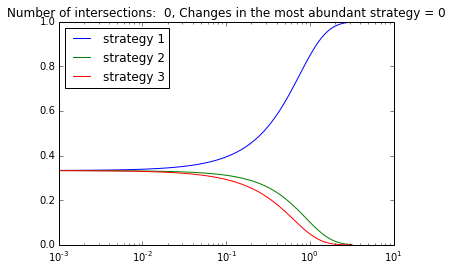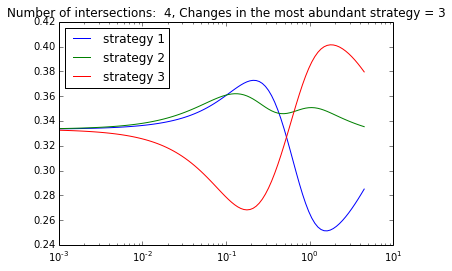# Extrapolating weak selection in Evolutionary Games¶

In :
%pylab inline

Populating the interactive namespace from numpy and matplotlib


This notebook assumes that ews.py and visualization.py are in the same folder of this notebook.

## Estimating the number of ranking changes¶

We need to specify some parameters to call the estimation procedure.

In :
!python ews.py

Traceback (most recent call last):
File "ews.py", line 298, in <module>
assert len(sys.argv) == 5, "You must specify the SEED, the NUMBER_OF_STRATEGIES " \
AssertionError: You must specify the SEED, the NUMBER_OF_STRATEGIES and the NUMBER_OF_REPETITIONS, and the DISTRIBUTION_TYPE 0 -- UNIFORM, 1 -- NORMAL


Let us call the estimation procedure with SEED=1234, 3 strategies, 50 samples and uniformly distributed games. Fifty samples will not give a quality estimate, but it is fine for demonstration purposes. This will take a couple of minutes...

In :
!python ews.py 1234 3 50 0

Files n_3_dist_0_seed_1234_count.pickle and ma_n_3_dist_0_seed_1234_count.pickle have been created


We can list the newly created files

In :
!ls -l *.pickle

-rw-rw-rw-  1 garcia  staff  54 Oct  4 10:46 ma_n_3_dist_0_seed_1234_count.pickle
-rw-rw-rw-  1 garcia  staff  62 Oct  4 10:46 n_3_dist_0_seed_1234_count.pickle


We can inspect the results by unpickling the new files.

In :
import pickle
filename_ranking_counts = 'n_3_dist_0_seed_1234_count.pickle'
filename_most_abundant_counts = 'ma_n_3_dist_0_seed_1234_count.pickle'

In :
ranking_changes = pickle.load(open(filename_ranking_counts))

In :
ranking_changes

Out:
Counter({0: 34, 1: 13, 2: 3})

In this paticular estimate, out of 50 samples, 34 have no ranking changes, 13 have one ranking change and 3 have two ranking changes.

In :
most_abundant_changes

Out:
Counter({0: 42, 1: 8})

In 8 out of 50 cases, the most abundant strategy changes once.

# Inside the sampling procedure¶

We first import ews.py to load all the function definitions.

In :
from ews import *


The first step in the estimation is to generate a random game. For this example, we use 3 strategies and uniformly distributed payoffs.

In :
number_of_strategies = 3
distribution_type = 0 # for uniform
a_random_game = random_game(number_of_strategies, distribution_type)
print a_random_game

[[ 0.89150299  0.18478255  0.43155487]
[ 0.0920854   0.80987834  0.04519806]
[ 0.88729132  0.56049353  0.20090459]]


Let us create a vector of selection intensities, in order to compute the abundance curves. Inside this process, for every intensity of selection we will compute the transition matrix of the markov chain that describes the imitation process, and then compute the stationary distribution.

We use the default limits in variables LEFT_LIMIT and RIGHT_LIMIT:

In :
'Intensity of selection interval = {}'.format((10**LEFT_LIMIT, 10**RIGHT_LIMIT))

Out:
'Intensity of selection interval = (0.001, 4.466835921509632)'
In :
intensity_vector = np.logspace(LEFT_LIMIT, RIGHT_LIMIT, num=100,
endpoint=True)


We create an auxliary matrix that contains the abundances vector across the values of our intensity vector.

Therefore, this matrix has as many columns as strategies in our game, and as many rows as values in intensity_vector

In :
population_size = 30
matrix = get_curves_truncating(intensity_vector, a_random_game,
population_size,
truncate=True)


What are the dimensions of matrix?

In :
matrix.shape

Out:
(96, 3)

Note that if matrix has lesss than 100 values, the graph has been truncated to avoid overflow. This happens when selection is strong enough and the stationary distribution becomes a singleton.

We can now count how many intersections are there for this case:

In :
count_intersections(matrix)

Out:
0

And how many changes in the most abundant strategy

In :
count_most_abundant_changes(matrix)

Out:
0

Now we use the function in visualization.py to build a graph.

In :
from visualization import plot_abundance

In :
plot_abundance(matrix, intensity_vector)You can re-run the cells up to here to generate another example.

## Using the functions judiciously¶

We can use the functions in ews.py, for instance, to replicate Figure 3A.

In :
figure_3a_game = np.array([[0.18,0.91,0.85],[0.11,0.59,0.78],[0.75,0.11,0.54]])

In :
intensity_vector = np.logspace(LEFT_LIMIT, RIGHT_LIMIT, num=100,
endpoint=True)

In :
auxiliary_matrix = get_curves_truncating(intensity_vector, figure_3a_game, population_size=30, truncate=True)

In :
plot_abundance(auxiliary_matrix, intensity_vector)## Unit Tests¶

We can also run the unit tests.

In :
!python tests.py

........
----------------------------------------------------------------------
Ran 8 tests in 2.885s

OK